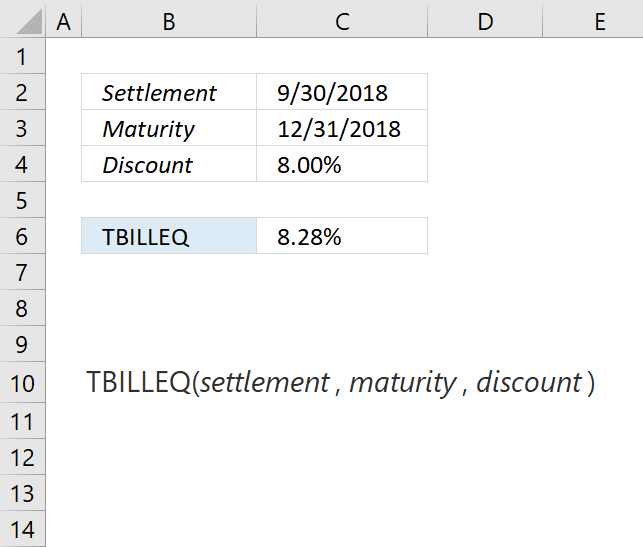Author: Oscar Cronquist Article last updated on December 11, 2018The TBILLPRICE function calculates the equivalent bond yield for a Treasury bill.

Formula in cell C6:

=TBILLEQ(C2,C3,C4)

### Excel Function Syntax

TBILLEQ(settlement, maturity, discount)

### Arguments

 settlement Required. The Treasury bill's settlement date which is the date after the issue date. maturity Required. The date when the security expires. discount Required. The Treasury bill's discount rate.

Treasury bills are issued at a discount from the face value, the interest paid is the face value - purchase price.

Keep in mind to use the DATE function if you enter dates in the function instead of using cell references.

For example,

=TBILLEQ(DATE(2018, 9, 30), DATE(2018, 12, 31), C4)

Date arguments are truncated to integers.

The TBILLEQ function returns:

• #VALUE! error if settlement or maturity is not a valid data type.
• #NUM! error if
• discount <=0 (zero)
• settlement > maturity, or if maturity is more than a year after the settlement

Calculation formula:

TBILLEQ = (365 * rate)/(360-(rate*DSM))

DSM = days between settlement to maturity ignoring maturity date that is more than a year after settlement.